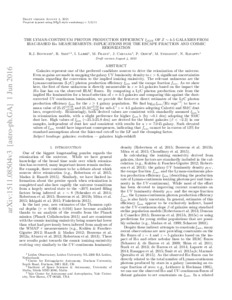# THE LYMAN-CONTINUUM PHOTON PRODUCTION EFFICIENCY xi(ION) OF z similar to 4-5 GALAXIES FROM IRAC-BASED H alpha MEASUREMENTS: IMPLICATIONS FOR THE ESCAPE FRACTION AND COSMIC REIONIZATION

Bouwens, RJ, Smit, R, Labbe, I, Franx, M, Caruana, J, Oesch, P, Stefanon, M and Rasappu, N (2016) THE LYMAN-CONTINUUM PHOTON PRODUCTION EFFICIENCY xi(ION) OF z similar to 4-5 GALAXIES FROM IRAC-BASED H alpha MEASUREMENTS: IMPLICATIONS FOR THE ESCAPE FRACTION AND COSMIC REIONIZATION. Astrophysical Journal, 831 (2). ISSN 0004-637XPreview
Text
1511.08504v3.pdf - Accepted Version

Galaxies represent one of the preferred candidate sources to drive the reionization of the universe. Even as gains are made in mapping the galaxy UV luminosity density to $z\gt 6$, significant uncertainties remain regarding the conversion to the implied ionizing emissivity. The relevant unknowns are the Lyman-continuum (LyC) photon production efficiency ${\xi }_{\mathrm{ion}}$ and the escape fraction f esc. As we show here, the first of these unknowns is directly measurable in z = 4–5 galaxies based on the impact the Hα line has on the observed IRAC fluxes. By computing a LyC photon production rate from the implied Hα luminosities for a broad selection of z = 4–5 galaxies and comparing this against the dust-corrected UV-continuum luminosities, we provide the first-ever direct estimates of the LyC photon production efficiency ${\xi }_{\mathrm{ion}}$ for the $z\geqslant 4$ galaxy population. We find $\,{\mathrm{log}}_{10}\,{\xi }_{\mathrm{ion}}/[\mathrm{Hz}\,{\mathrm{erg}}^{-1}]$ to have a mean value of ${25.27}_{-0.03}^{+0.03}$ and ${25.34}_{-0.02}^{+0.02}$ for sub-L* z = 4–5 galaxies adopting Calzetti and SMC dust laws, respectively. Reassuringly, both derived values are consistent with the standard assumed ${\xi }_{\mathrm{ion}}$'s in reionization models, with a slight preference for higher ${\xi }_{\mathrm{ion}}$'s (by ~0.1 dex) adopting the SMC dust law. High values of ${\xi }_{\mathrm{ion}}$ (~25.5–25.8 dex) are derived for the bluest galaxies ($\beta \lt -2.3$) in our samples, independent of dust law and consistent with results for a z = 7.045 galaxy. Such elevated values of ${\xi }_{\mathrm{ion}}$ would have important consequences, indicating that f esc cannot be in excess of 13% for standard assumptions about the faint-end cut-off to the LF and the clumping factor.View Item